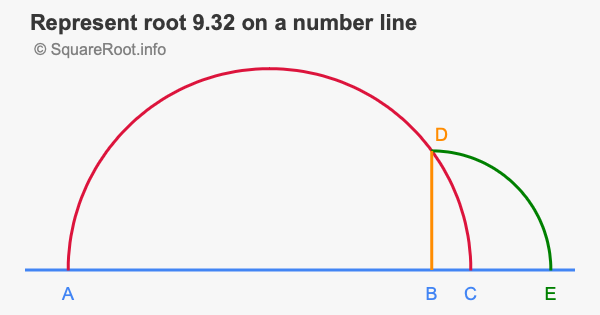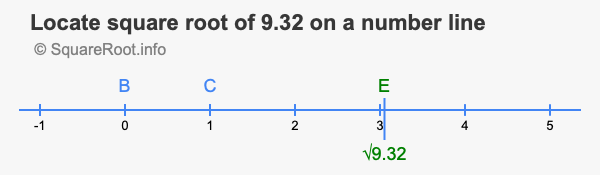Represent root 9.32 on a number lineHere we will show you step-by-step how to represent the square root of 9.32 on a number line geometrically. We recommend that you get your pencil, ruler, and compass ready so you can copy the steps on a piece of paper.

We have color coded the steps so it will be easy to follow along. We first show you our illustration of the finished product, and then explain step-by-step how we created it:Step 1)
Draw a line A B that is 9.32 in length, and then add 1 to C so that the distance from A to C is 10.32.

Step 2)
From the midpoint of A C on the line, use your compass and make a half circle from A to C.

Step 3)
Draw a vertical line up from B until it touches the red half circle you made in Step 2.

Step 4)
From B on the number line, use your compass and make a quarter circle from D to E.

The square root of 9.32 is the distance from B to E. To make things clearer, we have also created the illustration below showing where B, E, and the square root of 9.32 are located on the number line, labeled with numbers instead of the half circle, quarter circle and vertical line.The square root of 9.32 (√9.32) is approximately 3.05287, which is written in green on the number line above.

Square Root on a Number Line
Please enter another square root for us to represent geometrically on a number line.

Represent root  on a number line.

Represent root 9.33 on a number line
Here is the next square root on our list that we have an illustration for. Try to do it on your own first, and then check your answer here.